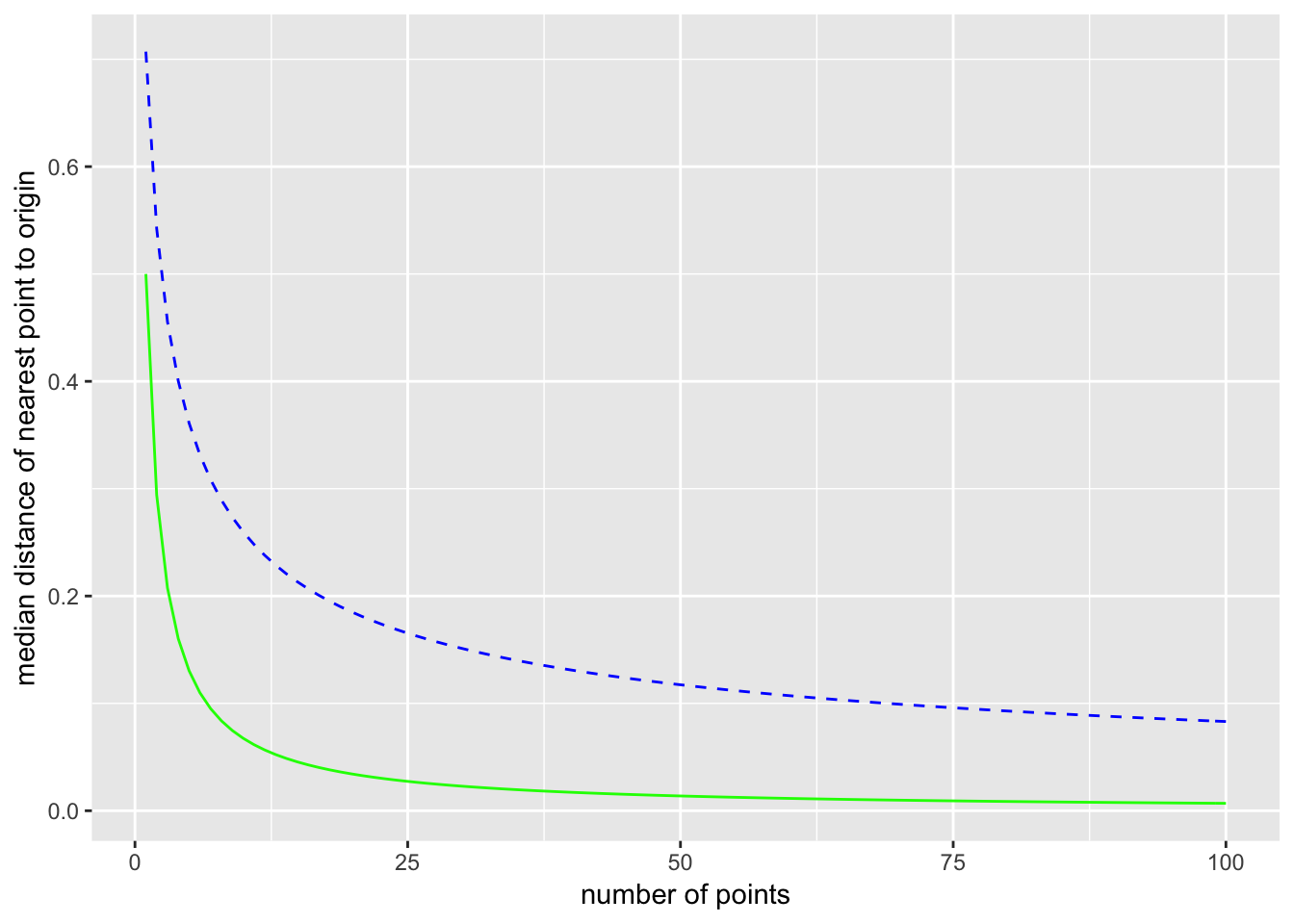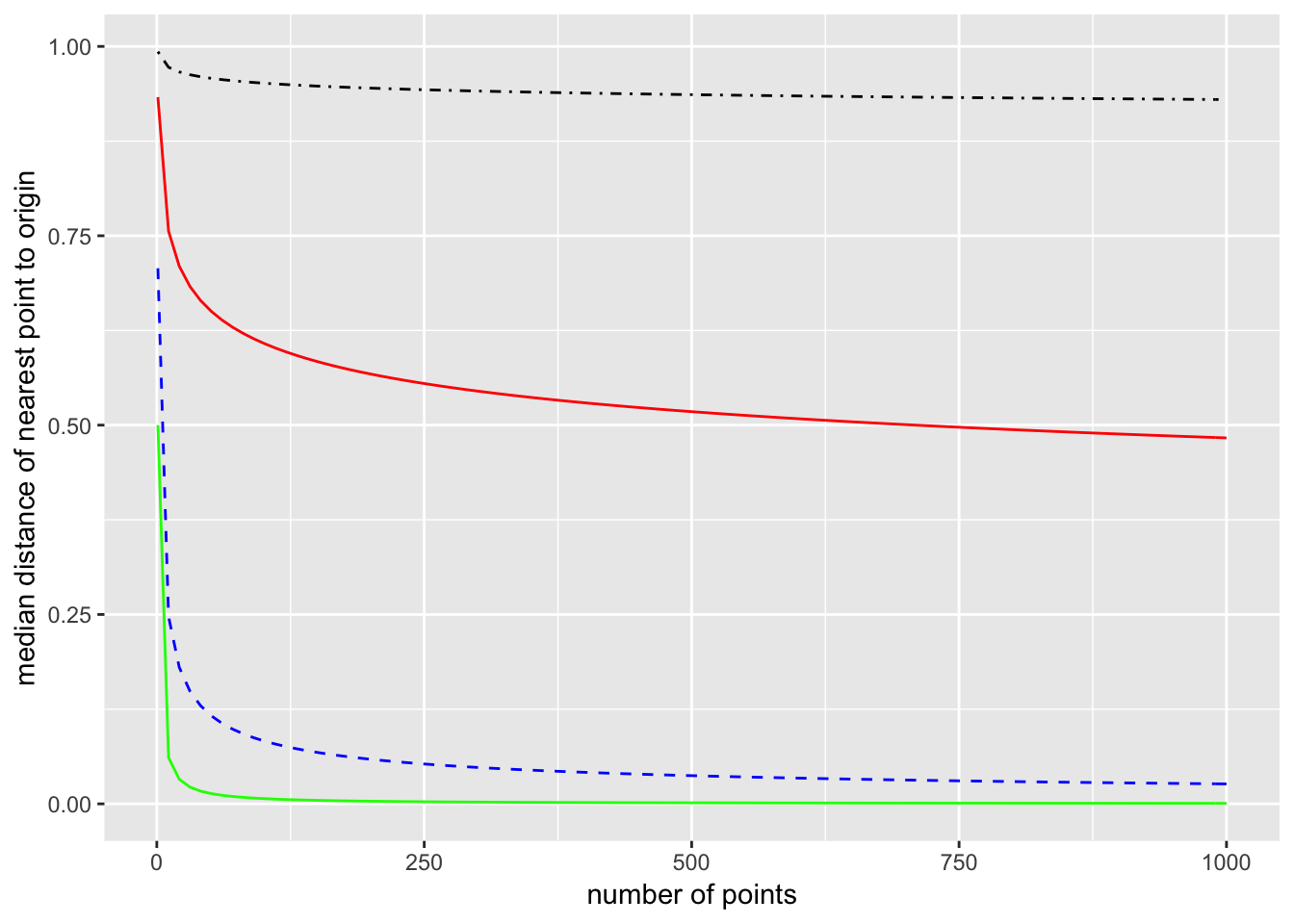# (Median) Distances in Uniform Distributions

Take a uniform distribution on $$[-1, 1]$$, so $$p(x) = 1/2$$. Pick a single point from this distribution. The probability that this point is within a distance $$m$$ from the origin is $$2m/2 = m$$. The probability that this point is not within a distance $$m$$ from the origin is then $$1-m$$.

Now consider picking $$N$$ points from the distribution. The probability that all $$N$$ points are further than $$m$$ from the origin is $$(1-m)^N$$. (Since $$m < 1$$, this number gets smaller as $$N$$ increases.) Now if we let $$m$$ mean the median distance from the origin to the nearest of the $$N$$ points, then by the definition of median $\text{Pr}(\text{all}\,N\, \text{points are futher than } m \text{ from the origin}) = \frac{1}{2} .$ Setting the two expressions equal $(1 - m)^N = \frac{1}{2},$ and solving for $$m$$ gives $m = 1 - \left( \frac{1}{2}\right)^{\frac{1}{N}} .$ For $$N = 10$$, $$m = 0.07$$, and for $$N = 100$$, $$m = 0.007$$. Of course, as $$N$$ goes to infinity, the median distance $$m$$ to the nearest point goes to zero.

Now expand this to the 2-dimensional unit ball with a uniform distribution. Here the probability of finding a point in any infinitesimal area element $$\text{d}A = \text{d}x\,\text{d}y$$ is the same for all area elements in the unit ball (a circle of radius 1). The area is $$\pi$$, so $$p(x,y) = 1/\pi$$. The probability of a single point from this uniform distribution being within a distance $$m$$ from the origin is the ratio of the area contained within radius $$m$$ to the total area: $\frac{\pi m^2}{\pi} = m^2.$ The probability of taking $$N$$ points and having none of them be within a distance $$m$$ of the origin is then $(1 - m^2)^N.$ Again let $$m$$ be the median distance to the nearest point, so we have the equation $(1 - m^2)^N = \frac{1}{2}.$ Solving for $$m$$ gives $m = \left( 1 - \left(\frac{1}{2}\right)^{\frac{1}{N}} \right)^{\frac{1}{2}}.$ For $$N = 10$$, $$m = 0.26$$, and for $$N = 100$$, $$m = 0.08$$. The median distance to the nearest point is larger in 2 dimensions than it is in one dimension. The distance still goes to zero as $$N$$ goes to infinity, but it does so more slowly.

Here is a plot comparing one and two dimensions (1D is solid green and 2D is dashed blue):This can be further generalized to $$p$$ dimensions. The volume of a $$p$$-dimensional ball of radius $$R$$ is $V_p (R) = \frac{\pi^{p/2}}{\Gamma(\frac{p}{2} +1)} R^p,$ and the constants will cancel out when taking ratios. So the probability that a single point chosen from a uniform distribution over the ball is closer than a distance $$m$$ from the origin is $$m^p$$. Proceeding as we did for one and two dimensions, the median distance of the closest point in $$p$$-dimensions is $m = \left(1 - \left(\frac{1}{2}\right)^{\frac{1}{N}}\right)^{\frac{1}{p}}.$ Here is a plot comparing dimensions 1, 2, 10, and 100. (1 is the bottom green line, 2 is the dashed blue line, 10 is the solid red line, and 100 is the dot-dashed black line.)The takeaway is that in high dimensions, you will probably have to go most of the way to the boundary before you encounter a single data point. If the dimension is $$p = 100$$, then you have to have samples of around $$10^{12}$$ points before the median distance from the origin to the nearest point is $$0.75$$.##### Landon Lehman
###### Data Scientist

My research interests include data science, statistics, physics, and applied math.